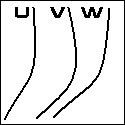Nomography Main > Standard Forms of the Third Class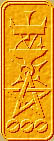ρ=Σ+Ψ
``` <!-- RegisterNomoPage("standardForms.html") PrintNomoHeader("Reference", "Antique Instruments"); PrintNomoPageNum() //--> ```

# Standard Forms of the Third Class

## i.e., Cheat Sheets

Note: If this "r=S+Y" is not composed of letters of the Greek alphabet, you probably have Netscape 6+ or Mozilla. You can enable the font with instructions found here.

These tables are taken from The Nomogram: The Theory And Practical Construction Of Computational Charts by H.J. Allcock and J.R. Jones (1946). They are for nomograms of equations with three variables (u,v,w). If you can reduce your equation to one resembling an entry in these tables you will save yourself an awful lot of work. Note that while these are only good for equations of three variables, it is possible to use them for a three variable component of a mult-variable nomogram.

If you cannot reduce your equation, I'm sorry, you are going to have to do it the hard way.

δ1, δ3, μ1, and μ3 are functional moduli (scaling values). In a spreadsheet you will alter these values by hand until the nomogram looks best.

The "Class" of a nomogram is the number of variables in the equation it solves for, all the nomograms on this page are of Class 3. The "Genus" of a nomogram is the number of functions in the equation minus the number of variables. So a nomogram of the equation f(u) - { { g(v) . f(w) - f(v) . g(w) } / { g(v) - g(w) } } = 0 has a Class of 3 (for u, v, and w) and a Genus of 2 (f(u), f(v), f(w), g(v), g(w) is five functions, minus three is Genus 2)

GENUS 0
f(u) + f(v) + f(w) = 0 Parallel Scale Nomogram U + V = W
Basic
Determinant
Constructional
Determinant
 -1 f(u) 1 0 -1/2 f(v) 1 1 f(w) 1
=0
 -δ1 μ1 . f(u) 1 0 -{{μ1 . μ3} / {μ1+μ3}} . f(v) 1 δ3 μ3 . f(w) 1
=0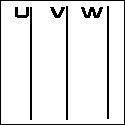where δ1331 or
 -δ1 { 1/δ3 } . f(u) 1 0 -{ 1 / { δ1 + δ3 }} . f(v) 1 δ3 { 1/δ1 } . f(w) 1

f(w) + f(v) . f(w) - k . f(v) - f(u) . f(v) = 0
Basic
Determinant
Constructional
Determinant
 0 f(u) 1 f(v) k . f(v) 1 1 f(w) 1
=0
 0 μ1 . f(u) 1 {δ3 . μ1 . f(v)} / {{μ1 - μ3}f(v) + μ3} {μ1 . μ3 . k . f(v)} / {{μ1 - μ3}f(v) + μ3} 1 δ3 μ3 . f(w) 1
=0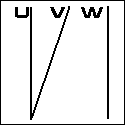f(v) - { f(u) / { f(u) + f(w) } } = 0
Basic
Determinant
Constructional
Determinant
 0 f(u) 1 f(v) 0 1 1 -f(w) 1
=0
 0 μ1 . f(u) 1 {δ3 . μ1 . f(v)} / {{μ1 - μ3}f(v) + μ3} 0 1 δ3 -μ3 . f(w) 1
=0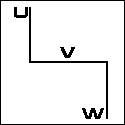f(v) - { f(u) / f(w) } = 0 The "N" or "Z" Nomogram U / W = V
Basic
Determinant
Constructional
Determinant
 0 f(u) 1 f(v) / { f(v) + 1} 0 1 1 -f(w) 1
=0
 0 μ1 . f(u) 1 {δ3 . μ1 { f(v) / { f(v)+1} } } / {{μ1 - μ3}{ f(v) / { f(v)+1} } + μ3} 0 1 δ3 -μ3 . f(w) 1
=0f(v) - { { f(u) . f(w) } / { f(u) + f(w) } } = 0 The Concurrent-scale nomogram
Basic
Determinant
Constructional
Determinant
 f(u) 0 1 f(v) f(v) 1 0 f(w) 1
=0
 δ1 . f(u) 0 1 δ1 . f(v) μ3 . f(v) 1 0 μ3 . f(w) 1
=0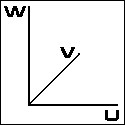f(u) + { { f(w){ f(v)+k1 }} / { { f(v) + k2 } - f(w)}} = 0
Basic
Determinant
Constructional
Determinant
 f(u) 0 1 f(v) + k1 f(v) + k2 1 0 f(w) 1
=0
 δ1 . f(u) 0 1 δ1{ f(v) + k1 } μ3{ f(v) + k2 } 1 0 μ3 . f(w) 1
=0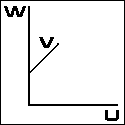f(u) = f(v) Expanded Two Variable Nomogram
Basic
Determinant
Constructional
Determinant
 -1 f(u) 1 0 0 1 1 -f(v) 1
=0
 -δ1 μ1 . f(u) 1 0 0 1 δ3 -μ3 . f(v) 1
=0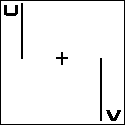where
δ1331

GENUS I
f(u) - f(v) + g(v){ f(w) - f(u)} = 0
Basic
Determinant
Constructional
Determinant
 0 f(u) 1 g(v) f(v) 1 1 f(w) 1
=0
 0 μ1 . f(u) 1 { δ3 . μ1 . g(v) } / { { μ1 - μ3 }g(v) + μ3 } { μ1 . μ3 . f(v) } / { { μ1 - μ3 }g(v) + μ3 } 1 δ3 μ3 . f(w) 1
=0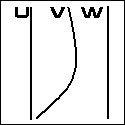f(u) . f(v) + g(v) . f(w) - f(w) . f(u) = 0
Basic
Determinant
Constructional
Determinant
 f(u) 0 1 g(v) f(v) 1 0 f(w) 1
=0
 δ1 . f(u) 0 1 δ1 . g(v) μ3 . f(v) 1 0 μ3 . f(w) 1
=0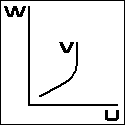GENUS II
f(u) - { { g(v) . f(w) - f(v) . g(w) } / { g(v) - g(w) } } = 0
Basic
Determinant
Constructional
Determinant
 0 f(u) 1 g(v) f(v) 1 g(w) f(w) 1
=0
 0 μ1 . f(u) 1 δ3 . g(v) μ1 . f(v) 1 δ3 . g(w) μ1 . f(w) 1
=0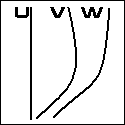GENUS III
g(u){ f(v) - f(w) } + g(v){ f(w) - f(u) } + g(w){ f(u) - f(v) } = 0
Basic
Determinant
Constructional
Determinant
 g(u) f(u) 1 g(v) f(v) 1 g(w) f(w) 1
=0
 δ3 . g(u) μ1 . f(u) 1 δ3 . g(v) μ1 . f(v) 1 δ3 . g(w) μ1 . f(w) 1
=0